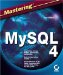# Aggregate Functions

Aggregate functions are functions that work on a group of data (meaning they can be used in a GROUP BY clause). If there is no GROUP BY clause, they will assume the entire result set is the group and return only one result. For the following examples, assume a simple table exists, as follows:

`mysql> SELECT * FROM table1; +--------+ | field1 | +--------+ |      4 | |     12 | |     12 | |     20 | +--------+ 4 rows in set (0.00 sec) `

### AVG

`AVG(expression)`

Returns the average value of the expressions in the group. Will return 0 if the expression is not numeric.

For example:

`mysql> SELECT AVG(field1) FROM table1; +-------------+ | AVG(field1) | +-------------+ |     12.0000 | +-------------+`

### BIT_AND

`BIT_AND(expression)`

Returns the bitwise AND of all bits in the expressions from the group (performed with 64-bit precision).

`mysql> SELECT BIT_AND(field1) FROM table1; +-----------------+ | BIT_AND(field1) | +-----------------+ |               4 | +-----------------+`

### BIT_OR

`BIT_OR(expession)`

Returns the bitwise OR of all bits in the expressions from the group (performed with 64-bit precision).

For example:

`mysql> SELECT BIT_OR(field1) FROM table1; +----------------+ | BIT_OR(field1) | +----------------+ |             28 | +----------------+`

### COUNT

`COUNT( [DISTINCT] expression1, [expression2])`

Returns the number of non-null values in the group.

If the expression is a field, returns the number of rows that don't contain null values in this field. COUNT(*) the number of all rows, null or not. The DISTINCT option returns the number of unique non-null values (or a combination, if more than one expression is used).

`mysql> SELECT COUNT(*) FROM table1; +----------+ | COUNT(*) | +----------+ |        4 | +----------+`

### MAX

`MAX(expression)`

Returns the largest value of the expressions in the group. The expression can be numeric or string.

For example:

`mysql> SELECT MAX(field1) FROM table1; +-------------+ | MAX(field1) | +-------------+ |          20 | +-------------+`

### MIN

`MIN(expression)`

Returns the smallest value of the expressions in the group. The expression can be numeric or string.

For example:

`mysql> SELECT MIN(field1) FROM table1; +-------------+ | MIN(field1) | +-------------+ |           4 | +-------------+ `

### STD

`STD(expression)`

Returns the standard deviation of the values in the expressions from the group.

For example:

`mysql> SELECT STD(field1) FROM table1; +-------------+ | STD(field1) | +-------------+ |      5.6569 | +-------------+`

### STDDEV

`STDDEV(expression)`

A synonym for the STD() function.

### SUM

`SUM(expression)`

Returns the smallest value of the expressions in the group or NULL if there are no rows. The expression can be numeric or string.

For example:

`mysql> SELECT SUM(field1) FROM table1; +-------------+ | MIN(field1) | +-------------+ |          48 | +-------------+`Mastering MySQL 4
ISBN: 0782141625
EAN: 2147483647
Year: 2003
Pages: 230
Authors: Ian Gilfillan